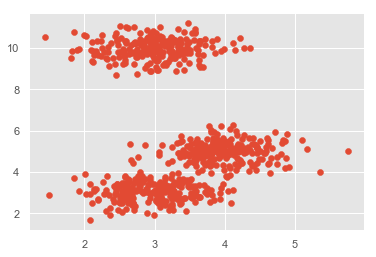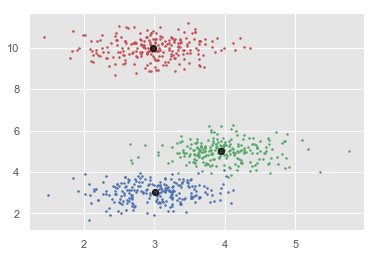# Clustering Algorithms - Mean Shift Algorithm

## Introduction to Mean-Shift Algorithm

As discussed earlier, it is another powerful clustering algorithm used in unsupervised learning. Unlike K-means clustering, it does not make any assumptions; hence it is a non-parametric algorithm.

Mean-shift algorithm basically assigns the datapoints to the clusters iteratively by shifting points towards the highest density of datapoints i.e. cluster centroid.

The difference between K-Means algorithm and Mean-Shift is that later one does not need to specify the number of clusters in advance because the number of clusters will be determined by the algorithm w.r.t data.

## Working of Mean-Shift Algorithm

We can understand the working of Mean-Shift clustering algorithm with the help of following steps −

• Step 1 − First, start with the data points assigned to a cluster of their own.

• Step 2 − Next, this algorithm will compute the centroids.

• Step 3 − In this step, location of new centroids will be updated.

• Step 4 − Now, the process will be iterated and moved to the higher density region.

• Step 5 − At last, it will be stopped once the centroids reach at position from where it cannot move further.

## Implementation in Python

It is a simple example to understand how Mean-Shift algorithm works. In this example, we are going to first generate 2D dataset containing 4 different blobs and after that will apply Mean-Shift algorithm to see the result.

%matplotlib inline
import numpy as np
from sklearn.cluster import MeanShift
import matplotlib.pyplot as plt
from matplotlib import style
style.use("ggplot")
from sklearn.datasets.samples_generator import make_blobs
centers = [[3,3,3],[4,5,5],[3,10,10]]
X, _ = make_blobs(n_samples = 700, centers = centers, cluster_std = 0.5)
plt.scatter(X[:,0],X[:,1])
plt.show()ms = MeanShift()
ms.fit(X)
labels = ms.labels_
cluster_centers = ms.cluster_centers_
print(cluster_centers)
n_clusters_ = len(np.unique(labels))
print("Estimated clusters:", n_clusters_)
colors = 10*['r.','g.','b.','c.','k.','y.','m.']
for i in range(len(X)):
plt.plot(X[i], X[i], colors[labels[i]], markersize = 3)
plt.scatter(cluster_centers[:,0],cluster_centers[:,1],
marker=".",color='k', s=20, linewidths = 5, zorder=10)
plt.show()


Output

[[ 2.98462798 9.9733794 10.02629344]
[ 3.94758484 4.99122771 4.99349433]
[ 3.00788996 3.03851268 2.99183033]]
Estimated clusters: 3The following are some advantages of Mean-Shift clustering algorithm −

• It does not need to make any model assumption as like in K-means or Gaussian mixture.

• It can also model the complex clusters which have nonconvex shape.

• It only needs one parameter named bandwidth which automatically determines the number of clusters.

• There is no issue of local minima as like in K-means.

• No problem generated from outliers.